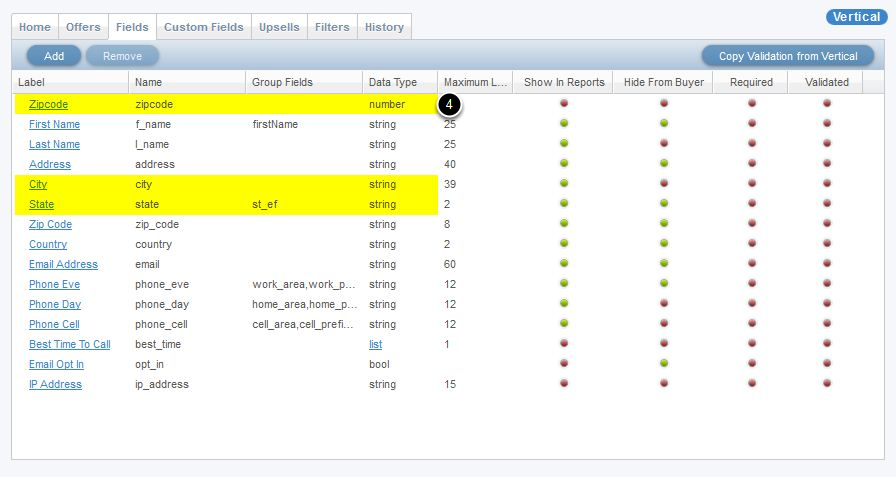# How to Setup Condition Formulas on Buyer Contracts

- What condition formulas do

- Zip, City & State Condition Formulas on Buyer Contracts

What condition formulas do

Condition formulas refine the lead fields that can be sold to the Buyer Contract.

Vertical fieldsHere is a reference to the fields that we will be validating with our condition formulas. As you can see two are numbers and one is a string data type.

Below are 3 examples of condition formulas.1. This formula will evaluate whether the zip code (represented by the token #zipcode#) is equal to "55555".  Because the data type of the zip code field is a "number", the SQL formula used for this condition formula does not need single quotes around the token as we do for string values. If the condition formula equates to "True" then that parameter and value will be passed to the buyer on the post.

This next example (#2) depicts how your condition formula does not need to refer to the field you're evaluating.

2. This formula will evaluate whether the zip code is equal to "55555". If the formula equates to "True" then the City field will be passed to the Buyer.  If you wanted, you could add multiple different tokens in here to evaluate and receive a True/False response.
3. This condition formula is similar to the previous ones but is evaluating a string data type field, and requires wrapping both the token and the value we're validating against in single quotes.

Tips:

• Number data types do not need single quotes
• All other data types (including string, bool, date, and list) require single quotes around the field token and the value

Did you find it helpful? Yes No

Send feedback